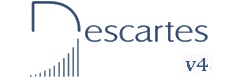First and Second Year of Secondary Education INDEX Introduction Objectives Integers 1- The addition of integers 2- The properties of addition 3- Additions 4- Additions 5- Additions 6- Additions 7- Additions 8- Additions 9- Additions 10- Additions 1- The additive inverse or opposite of a number 2-The subtraction of integers
Operations with integers I
INTRODUCTION
In this unit, we revise the basic ideas of integers and we use them to practise the following operations: sums and subtractions.
Before starting this unit, students should have studied integers in class in the traditional way. These activities can be used as a complement to reinforce and understand the concepts and the operations better.

The numbers that appear in each window are generated at random. This allows you to use the window indefinitely and practise a lot of different exercises. Numbers usually consist of only one digit so the calculations can easily be done mentally.
Magic squares with sums and multiplications are used in the exercises, however the main objective of this unit is to learn things about integers.

OBJECTIVES
• To identify values which are integers.
• To represent graphically several integers on a straight line.
• To order the value of several integers.Spanish Ministry of Education. Year 2007